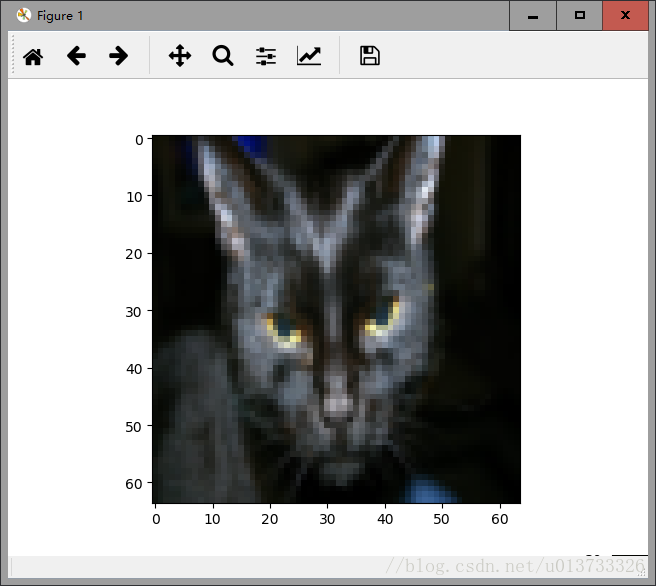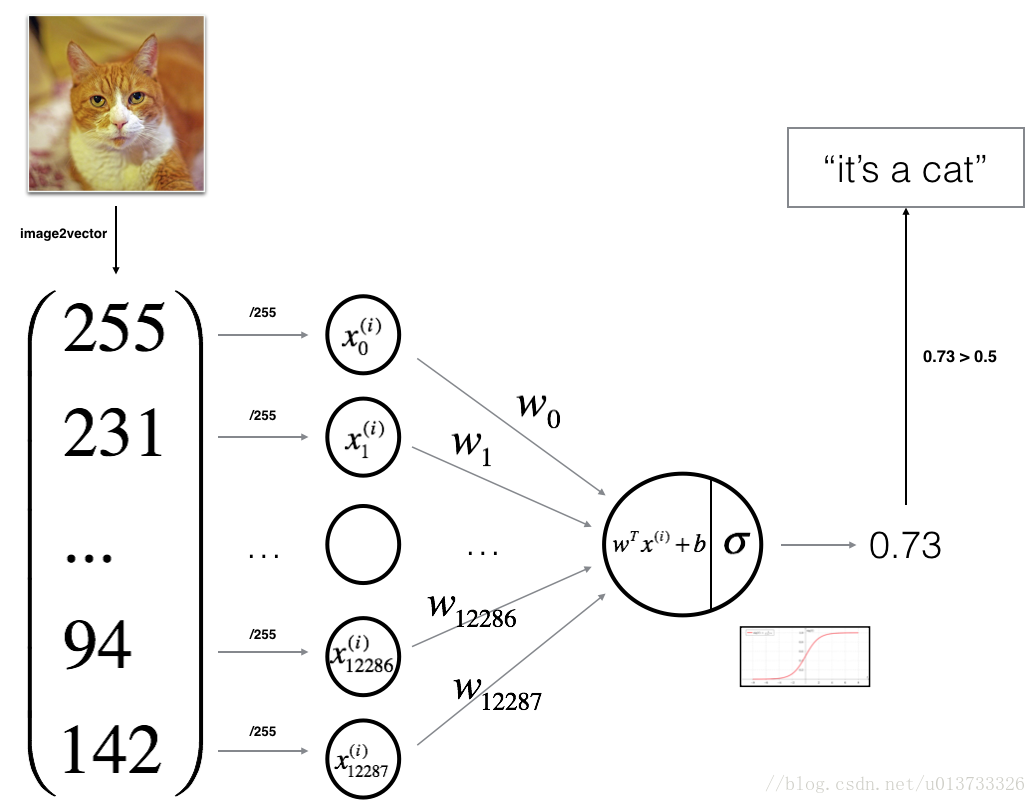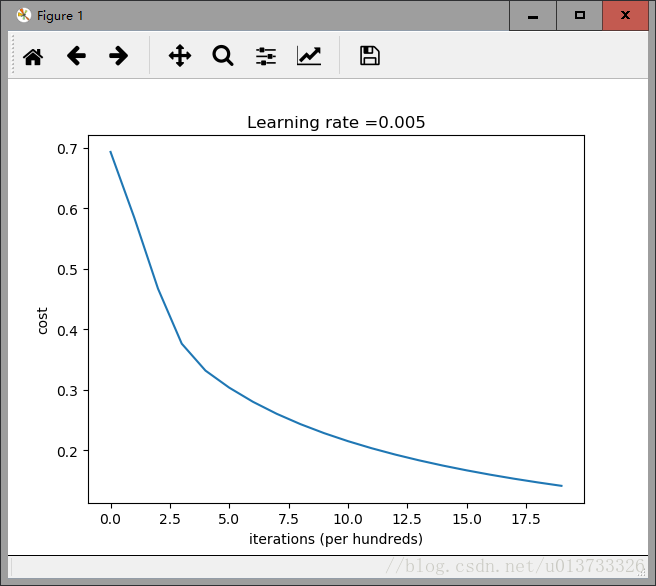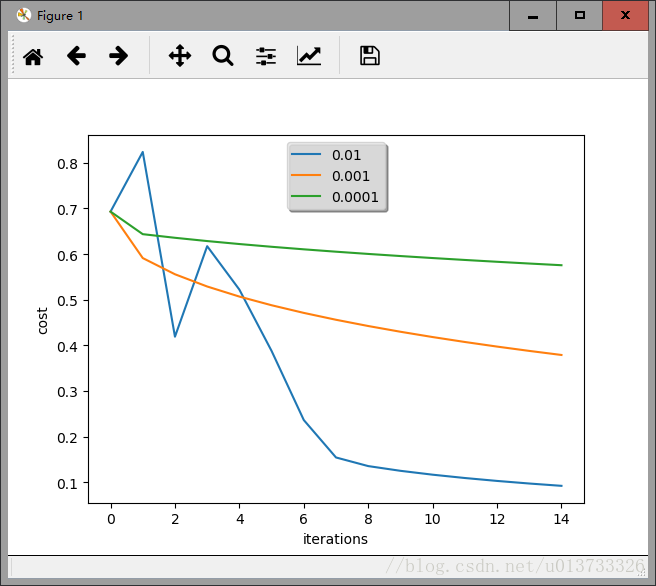# 【吴恩达课后编程作业】Course 1 - 神经网络和深度学习 - 第二周作业 - 具有神经网络思维的Logistic回归

【博主使用的python版本：3.6.2】

• numpy ：是用Python进行科学计算的基本软件包。
• h5py：是与H5文件中存储的数据集进行交互的常用软件包。
• matplotlib：是一个著名的库，用于在Python中绘制图表。
• lr_utils ：在本文的资料包里，一个加载资料包里面的数据的简单功能的库。

import numpy as np
import matplotlib.pyplot as plt
import h5py
from lr_utils import load_dataset



lr_utils.py代码如下，你也可以自行打开它查看：

import numpy as np
import h5py

def load_dataset():
train_dataset = h5py.File('datasets/train_catvnoncat.h5', "r")
train_set_x_orig = np.array(train_dataset["train_set_x"][:]) # your train set features
train_set_y_orig = np.array(train_dataset["train_set_y"][:]) # your train set labels

test_dataset = h5py.File('datasets/test_catvnoncat.h5', "r")
test_set_x_orig = np.array(test_dataset["test_set_x"][:]) # your test set features
test_set_y_orig = np.array(test_dataset["test_set_y"][:]) # your test set labels

classes = np.array(test_dataset["list_classes"][:]) # the list of classes

train_set_y_orig = train_set_y_orig.reshape((1, train_set_y_orig.shape))
test_set_y_orig = test_set_y_orig.reshape((1, test_set_y_orig.shape))

return train_set_x_orig, train_set_y_orig, test_set_x_orig, test_set_y_orig, classes


• train_set_x_orig ：保存的是训练集里面的图像数据（本训练集有209张64x64的图像）。
• train_set_y_orig ：保存的是训练集的图像对应的分类值（【0 | 1】，0表示不是猫，1表示是猫）。
• test_set_x_orig ：保存的是测试集里面的图像数据（本训练集有50张64x64的图像）。
• test_set_y_orig ： 保存的是测试集的图像对应的分类值（【0 | 1】，0表示不是猫，1表示是猫）。
• classes ： 保存的是以bytes类型保存的两个字符串数据，数据为：[b’non-cat’ b’cat’]。

train_set_x_orig , train_set_y , test_set_x_orig , test_set_y , classes = load_dataset()


index = 25
plt.imshow(train_set_x_orig[index])
#print("train_set_y=" + str(train_set_y)) #你也可以看一下训练集里面的标签是什么样的。#打印出当前的训练标签值
#使用np.squeeze的目的是压缩维度，【未压缩】train_set_y[:,index]的值为 , 【压缩后】np.squeeze(train_set_y[:,index])的值为1
#print("【使用np.squeeze：" + str(np.squeeze(train_set_y[:,index])) + "，不使用np.squeeze： " + str(train_set_y[:,index]) + "】")
#只有压缩后的值才能进行解码操作
print("y=" + str(train_set_y[:,index]) + ", it's a " + classes[np.squeeze(train_set_y[:,index])].decode("utf-8") + "' picture")


• m_train ：训练集里图片的数量。
• m_test ：测试集里图片的数量。
• num_px ： 训练、测试集里面的图片的宽度和高度（均为64x64）。

m_train = train_set_y.shape #训练集里图片的数量。
m_test = test_set_y.shape #测试集里图片的数量。
num_px = train_set_x_orig.shape #训练、测试集里面的图片的宽度和高度（均为64x64）。

#现在看一看我们加载的东西的具体情况
print ("训练集的数量: m_train = " + str(m_train))
print ("测试集的数量 : m_test = " + str(m_test))
print ("每张图片的宽/高 : num_px = " + str(num_px))
print ("每张图片的大小 : (" + str(num_px) + ", " + str(num_px) + ", 3)")
print ("训练集_图片的维数 : " + str(train_set_x_orig.shape))
print ("训练集_标签的维数 : " + str(train_set_y.shape))
print ("测试集_图片的维数: " + str(test_set_x_orig.shape))
print ("测试集_标签的维数: " + str(test_set_y.shape))


训练集的数量: m_train = 209



#X_flatten = X.reshape(X.shape ，-1).T ＃X.T是X的转置
#将训练集的维度降低并转置。
train_set_x_flatten  = train_set_x_orig.reshape(train_set_x_orig.shape,-1).T
#将测试集的维度降低并转置。
test_set_x_flatten = test_set_x_orig.reshape(test_set_x_orig.shape, -1).T


print ("训练集降维最后的维度： " + str(train_set_x_flatten.shape))
print ("训练集_标签的维数 : " + str(train_set_y.shape))
print ("测试集降维之后的维度: " + str(test_set_x_flatten.shape))
print ("测试集_标签的维数 : " + str(test_set_y.shape))


训练集降维最后的维度： (12288, 209)



train_set_x = train_set_x_flatten / 255
test_set_x = test_set_x_flatten / 2551. 定义模型结构（例如输入特征的数量）

2. 初始化模型的参数

3. 循环：

3.1 计算当前损失（正向传播）

3.2 计算当前梯度（反向传播）

3.3 更新参数（梯度下降）

def sigmoid(z):
"""
参数：
z  - 任何大小的标量或numpy数组。

返回：
s  -  sigmoid（z）
"""
s = 1 / (1 + np.exp(-z))
return s


#测试sigmoid()
print("====================测试sigmoid====================")
print ("sigmoid(0) = " + str(sigmoid(0)))
print ("sigmoid(9.2) = " + str(sigmoid(9.2)))


====================测试sigmoid====================
sigmoid(0) = 0.5
sigmoid(9.2) = 0.999898970806


def initialize_with_zeros(dim):
"""
此函数为w创建一个维度为（dim，1）的0向量，并将b初始化为0。

参数：
dim  - 我们想要的w矢量的大小（或者这种情况下的参数数量）

返回：
w  - 维度为（dim，1）的初始化向量。
b  - 初始化的标量（对应于偏差）
"""
w = np.zeros(shape = (dim,1))
b = 0
#使用断言来确保我要的数据是正确的
assert(w.shape == (dim, 1)) #w的维度是(dim,1)
assert(isinstance(b, float) or isinstance(b, int)) #b的类型是float或者是int

return (w , b)


def propagate(w, b, X, Y):
"""
实现前向和后向传播的成本函数及其梯度。
参数：
w  - 权重，大小不等的数组（num_px * num_px * 3，1）
b  - 偏差，一个标量
X  - 矩阵类型为（num_px * num_px * 3，训练数量）
Y  - 真正的“标签”矢量（如果非猫则为0，如果是猫则为1），矩阵维度为(1,训练数据数量)

返回：
cost- 逻辑回归的负对数似然成本
dw  - 相对于w的损失梯度，因此与w相同的形状
db  - 相对于b的损失梯度，因此与b的形状相同
"""
m = X.shape

#正向传播
A = sigmoid(np.dot(w.T,X) + b) #计算激活值，请参考公式2。
cost = (- 1 / m) * np.sum(Y * np.log(A) + (1 - Y) * (np.log(1 - A))) #计算成本，请参考公式3和4。

#反向传播
dw = (1 / m) * np.dot(X, (A - Y).T) #请参考视频中的偏导公式。
db = (1 / m) * np.sum(A - Y) #请参考视频中的偏导公式。

#使用断言确保我的数据是正确的
assert(dw.shape == w.shape)
assert(db.dtype == float)
cost = np.squeeze(cost)
assert(cost.shape == ())

#创建一个字典，把dw和db保存起来。
grads = {
"dw": dw,
"db": db
}
return (grads , cost)


#测试一下propagate
print("====================测试propagate====================")
#初始化一些参数
w, b, X, Y = np.array([, ]), 2, np.array([[1,2], [3,4]]), np.array([[1, 0]])
grads, cost = propagate(w, b, X, Y)
print ("dw = " + str(grads["dw"]))
print ("db = " + str(grads["db"]))
print ("cost = " + str(cost))


====================测试propagate====================
dw = [[ 0.99993216]
[ 1.99980262]]
db = 0.499935230625
cost = 6.00006477319


def optimize(w , b , X , Y , num_iterations , learning_rate , print_cost = False):
"""
此函数通过运行梯度下降算法来优化w和b

参数：
w  - 权重，大小不等的数组（num_px * num_px * 3，1）
b  - 偏差，一个标量
X  - 维度为（num_px * num_px * 3，训练数据的数量）的数组。
Y  - 真正的“标签”矢量（如果非猫则为0，如果是猫则为1），矩阵维度为(1,训练数据的数量)
num_iterations  - 优化循环的迭代次数
learning_rate  - 梯度下降更新规则的学习率
print_cost  - 每100步打印一次损失值

返回：
params  - 包含权重w和偏差b的字典
grads  - 包含权重和偏差相对于成本函数的梯度的字典
成本 - 优化期间计算的所有成本列表，将用于绘制学习曲线。

提示：
我们需要写下两个步骤并遍历它们：
1）计算当前参数的成本和梯度，使用propagate（）。
2）使用w和b的梯度下降法则更新参数。
"""

costs = []

for i in range(num_iterations):

grads, cost = propagate(w, b, X, Y)

dw = grads["dw"]
db = grads["db"]

w = w - learning_rate * dw
b = b - learning_rate * db

#记录成本
if i % 100 == 0:
costs.append(cost)
#打印成本数据
if (print_cost) and (i % 100 == 0):
print("迭代的次数: %i ， 误差值： %f" % (i,cost))

params  = {
"w" : w,
"b" : b }
grads = {
"dw": dw,
"db": db }
return (params , grads , costs)


#测试optimize
print("====================测试optimize====================")
w, b, X, Y = np.array([, ]), 2, np.array([[1,2], [3,4]]), np.array([[1, 0]])
params , grads , costs = optimize(w , b , X , Y , num_iterations=100 , learning_rate = 0.009 , print_cost = False)
print ("w = " + str(params["w"]))
print ("b = " + str(params["b"]))
print ("dw = " + str(grads["dw"]))
print ("db = " + str(grads["db"]))


====================测试optimize====================
w = [[ 0.1124579 ]
[ 0.23106775]]
b = 1.55930492484
dw = [[ 0.90158428]
[ 1.76250842]]
db = 0.430462071679


optimize函数会输出已学习的w和b的值，我们可以使用w和b来预测数据集X的标签。

1. 计算 Y ^ = A = σ ( w T X + b ) \hat{Y} = A = \sigma(w^T X + b)

2. 将a的值变为0（如果激活值<= 0.5）或者为1（如果激活值> 0.5），

def predict(w , b , X ):
"""
使用学习逻辑回归参数logistic （w，b）预测标签是0还是1，

参数：
w  - 权重，大小不等的数组（num_px * num_px * 3，1）
b  - 偏差，一个标量
X  - 维度为（num_px * num_px * 3，训练数据的数量）的数据

返回：
Y_prediction  - 包含X中所有图片的所有预测【0 | 1】的一个numpy数组（向量）

"""

m  = X.shape #图片的数量
Y_prediction = np.zeros((1,m))
w = w.reshape(X.shape,1)

#计预测猫在图片中出现的概率
A = sigmoid(np.dot(w.T , X) + b)
for i in range(A.shape):
#将概率a [0，i]转换为实际预测p [0，i]
Y_prediction[0,i] = 1 if A[0,i] > 0.5 else 0
#使用断言
assert(Y_prediction.shape == (1,m))

return Y_prediction


#测试predict
print("====================测试predict====================")
w, b, X, Y = np.array([, ]), 2, np.array([[1,2], [3,4]]), np.array([[1, 0]])
print("predictions = " + str(predict(w, b, X)))


def model(X_train , Y_train , X_test , Y_test , num_iterations = 2000 , learning_rate = 0.5 , print_cost = False):
"""
通过调用之前实现的函数来构建逻辑回归模型

参数：
X_train  - numpy的数组,维度为（num_px * num_px * 3，m_train）的训练集
Y_train  - numpy的数组,维度为（1，m_train）（矢量）的训练标签集
X_test   - numpy的数组,维度为（num_px * num_px * 3，m_test）的测试集
Y_test   - numpy的数组,维度为（1，m_test）的（向量）的测试标签集
num_iterations  - 表示用于优化参数的迭代次数的超参数
learning_rate  - 表示optimize（）更新规则中使用的学习速率的超参数
print_cost  - 设置为true以每100次迭代打印成本

返回：
d  - 包含有关模型信息的字典。
"""
w , b = initialize_with_zeros(X_train.shape)

parameters , grads , costs = optimize(w , b , X_train , Y_train,num_iterations , learning_rate , print_cost)

#从字典“参数”中检索参数w和b
w , b = parameters["w"] , parameters["b"]

#预测测试/训练集的例子
Y_prediction_test = predict(w , b, X_test)
Y_prediction_train = predict(w , b, X_train)

#打印训练后的准确性
print("训练集准确性："  , format(100 - np.mean(np.abs(Y_prediction_train - Y_train)) * 100) ,"%")
print("测试集准确性："  , format(100 - np.mean(np.abs(Y_prediction_test - Y_test)) * 100) ,"%")

d = {
"costs" : costs,
"Y_prediction_test" : Y_prediction_test,
"Y_prediciton_train" : Y_prediction_train,
"w" : w,
"b" : b,
"learning_rate" : learning_rate,
"num_iterations" : num_iterations }
return d


print("====================测试model====================")
#这里加载的是真实的数据，请参见上面的代码部分。
d = model(train_set_x, train_set_y, test_set_x, test_set_y, num_iterations = 2000, learning_rate = 0.005, print_cost = True)


====================测试model====================



#绘制图
costs = np.squeeze(d['costs'])
plt.plot(costs)
plt.ylabel('cost')
plt.xlabel('iterations (per hundreds)')
plt.title("Learning rate =" + str(d["learning_rate"]))
plt.show()learning_rates = [0.01, 0.001, 0.0001]
models = {}
for i in learning_rates:
print ("learning rate is: " + str(i))
models[str(i)] = model(train_set_x, train_set_y, test_set_x, test_set_y, num_iterations = 1500, learning_rate = i, print_cost = False)
print ('\n' + "-------------------------------------------------------" + '\n')

for i in learning_rates:
plt.plot(np.squeeze(models[str(i)]["costs"]), label= str(models[str(i)]["learning_rate"]))

plt.ylabel('cost')
plt.xlabel('iterations')

legend = plt.legend(loc='upper center', shadow=True)
frame = legend.get_frame()
frame.set_facecolor('0.90')
plt.show()


learning rate is: 0.01

-------------------------------------------------------

learning rate is: 0.001

-------------------------------------------------------

learning rate is: 0.0001

-------------------------------------------------------**【完整代码】: **

# -*- coding: utf-8 -*-
"""
Created on Wed Mar 21 17:25:30 2018

@author: Oscar
"""

import numpy as np
import matplotlib.pyplot as plt
import h5py
from lr_utils import load_dataset

train_set_x_orig , train_set_y , test_set_x_orig , test_set_y , classes = load_dataset()

m_train = train_set_y.shape #训练集里图片的数量。
m_test = test_set_y.shape #测试集里图片的数量。
num_px = train_set_x_orig.shape #训练、测试集里面的图片的宽度和高度（均为64x64）。

#现在看一看我们加载的东西的具体情况
print ("训练集的数量: m_train = " + str(m_train))
print ("测试集的数量 : m_test = " + str(m_test))
print ("每张图片的宽/高 : num_px = " + str(num_px))
print ("每张图片的大小 : (" + str(num_px) + ", " + str(num_px) + ", 3)")
print ("训练集_图片的维数 : " + str(train_set_x_orig.shape))
print ("训练集_标签的维数 : " + str(train_set_y.shape))
print ("测试集_图片的维数: " + str(test_set_x_orig.shape))
print ("测试集_标签的维数: " + str(test_set_y.shape))

#将训练集的维度降低并转置。
train_set_x_flatten  = train_set_x_orig.reshape(train_set_x_orig.shape,-1).T
#将测试集的维度降低并转置。
test_set_x_flatten = test_set_x_orig.reshape(test_set_x_orig.shape, -1).T

print ("训练集降维最后的维度： " + str(train_set_x_flatten.shape))
print ("训练集_标签的维数 : " + str(train_set_y.shape))
print ("测试集降维之后的维度: " + str(test_set_x_flatten.shape))
print ("测试集_标签的维数 : " + str(test_set_y.shape))

train_set_x = train_set_x_flatten / 255
test_set_x = test_set_x_flatten / 255

def sigmoid(z):
"""
参数：
z  - 任何大小的标量或numpy数组。

返回：
s  -  sigmoid（z）
"""
s = 1 / (1 + np.exp(-z))
return s

def initialize_with_zeros(dim):
"""
此函数为w创建一个维度为（dim，1）的0向量，并将b初始化为0。

参数：
dim  - 我们想要的w矢量的大小（或者这种情况下的参数数量）

返回：
w  - 维度为（dim，1）的初始化向量。
b  - 初始化的标量（对应于偏差）
"""
w = np.zeros(shape = (dim,1))
b = 0
#使用断言来确保我要的数据是正确的
assert(w.shape == (dim, 1)) #w的维度是(dim,1)
assert(isinstance(b, float) or isinstance(b, int)) #b的类型是float或者是int

return (w , b)

def propagate(w, b, X, Y):
"""
实现前向和后向传播的成本函数及其梯度。
参数：
w  - 权重，大小不等的数组（num_px * num_px * 3，1）
b  - 偏差，一个标量
X  - 矩阵类型为（num_px * num_px * 3，训练数量）
Y  - 真正的“标签”矢量（如果非猫则为0，如果是猫则为1），矩阵维度为(1,训练数据数量)

返回：
cost- 逻辑回归的负对数似然成本
dw  - 相对于w的损失梯度，因此与w相同的形状
db  - 相对于b的损失梯度，因此与b的形状相同
"""
m = X.shape

#正向传播
A = sigmoid(np.dot(w.T,X) + b) #计算激活值，请参考公式2。
cost = (- 1 / m) * np.sum(Y * np.log(A) + (1 - Y) * (np.log(1 - A))) #计算成本，请参考公式3和4。

#反向传播
dw = (1 / m) * np.dot(X, (A - Y).T) #请参考视频中的偏导公式。
db = (1 / m) * np.sum(A - Y) #请参考视频中的偏导公式。

#使用断言确保我的数据是正确的
assert(dw.shape == w.shape)
assert(db.dtype == float)
cost = np.squeeze(cost)
assert(cost.shape == ())

#创建一个字典，把dw和db保存起来。
grads = {
"dw": dw,
"db": db
}
return (grads , cost)

def optimize(w , b , X , Y , num_iterations , learning_rate , print_cost = False):
"""
此函数通过运行梯度下降算法来优化w和b

参数：
w  - 权重，大小不等的数组（num_px * num_px * 3，1）
b  - 偏差，一个标量
X  - 维度为（num_px * num_px * 3，训练数据的数量）的数组。
Y  - 真正的“标签”矢量（如果非猫则为0，如果是猫则为1），矩阵维度为(1,训练数据的数量)
num_iterations  - 优化循环的迭代次数
learning_rate  - 梯度下降更新规则的学习率
print_cost  - 每100步打印一次损失值

返回：
params  - 包含权重w和偏差b的字典
grads  - 包含权重和偏差相对于成本函数的梯度的字典
成本 - 优化期间计算的所有成本列表，将用于绘制学习曲线。

提示：
我们需要写下两个步骤并遍历它们：
1）计算当前参数的成本和梯度，使用propagate（）。
2）使用w和b的梯度下降法则更新参数。
"""

costs = []

for i in range(num_iterations):

grads, cost = propagate(w, b, X, Y)

dw = grads["dw"]
db = grads["db"]

w = w - learning_rate * dw
b = b - learning_rate * db

#记录成本
if i % 100 == 0:
costs.append(cost)
#打印成本数据
if (print_cost) and (i % 100 == 0):
print("迭代的次数: %i ， 误差值： %f" % (i,cost))

params  = {
"w" : w,
"b" : b }
grads = {
"dw": dw,
"db": db }
return (params , grads , costs)

def predict(w , b , X ):
"""
使用学习逻辑回归参数logistic （w，b）预测标签是0还是1，

参数：
w  - 权重，大小不等的数组（num_px * num_px * 3，1）
b  - 偏差，一个标量
X  - 维度为（num_px * num_px * 3，训练数据的数量）的数据

返回：
Y_prediction  - 包含X中所有图片的所有预测【0 | 1】的一个numpy数组（向量）

"""

m  = X.shape #图片的数量
Y_prediction = np.zeros((1,m))
w = w.reshape(X.shape,1)

#计预测猫在图片中出现的概率
A = sigmoid(np.dot(w.T , X) + b)
for i in range(A.shape):
#将概率a [0，i]转换为实际预测p [0，i]
Y_prediction[0,i] = 1 if A[0,i] > 0.5 else 0
#使用断言
assert(Y_prediction.shape == (1,m))

return Y_prediction

def model(X_train , Y_train , X_test , Y_test , num_iterations = 2000 , learning_rate = 0.5 , print_cost = False):
"""
通过调用之前实现的函数来构建逻辑回归模型

参数：
X_train  - numpy的数组,维度为（num_px * num_px * 3，m_train）的训练集
Y_train  - numpy的数组,维度为（1，m_train）（矢量）的训练标签集
X_test   - numpy的数组,维度为（num_px * num_px * 3，m_test）的测试集
Y_test   - numpy的数组,维度为（1，m_test）的（向量）的测试标签集
num_iterations  - 表示用于优化参数的迭代次数的超参数
learning_rate  - 表示optimize（）更新规则中使用的学习速率的超参数
print_cost  - 设置为true以每100次迭代打印成本

返回：
d  - 包含有关模型信息的字典。
"""
w , b = initialize_with_zeros(X_train.shape)

parameters , grads , costs = optimize(w , b , X_train , Y_train,num_iterations , learning_rate , print_cost)

#从字典“参数”中检索参数w和b
w , b = parameters["w"] , parameters["b"]

#预测测试/训练集的例子
Y_prediction_test = predict(w , b, X_test)
Y_prediction_train = predict(w , b, X_train)

#打印训练后的准确性
print("训练集准确性："  , format(100 - np.mean(np.abs(Y_prediction_train - Y_train)) * 100) ,"%")
print("测试集准确性："  , format(100 - np.mean(np.abs(Y_prediction_test - Y_test)) * 100) ,"%")

d = {
"costs" : costs,
"Y_prediction_test" : Y_prediction_test,
"Y_prediciton_train" : Y_prediction_train,
"w" : w,
"b" : b,
"learning_rate" : learning_rate,
"num_iterations" : num_iterations }
return d

d = model(train_set_x, train_set_y, test_set_x, test_set_y, num_iterations = 2000, learning_rate = 0.005, print_cost = True)

#绘制图
costs = np.squeeze(d['costs'])
plt.plot(costs)
plt.ylabel('cost')
plt.xlabel('iterations (per hundreds)')
plt.title("Learning rate =" + str(d["learning_rate"]))
plt.show()


11-0973012-033610
04-0548万+
10-257万+
07-261280
09-20231
09-231884
06-161660
02-025411
08-164571
10-10575
01-08
12-2812万+
04-129万+
09-254849
04-231万+
©️2020 CSDN 皮肤主题: 大白 设计师:CSDN官方博客点击重新获取扫码支付1.余额是钱包充值的虚拟货币，按照1:1的比例进行支付金额的抵扣。
2.余额无法直接购买下载，可以购买VIP、C币套餐、付费专栏及课程。余额充值